# ggplot2绘制高频时间序列

Posted by Elsa.Liu & Bruce Zhao on June 6, 2016

## 转换成xts格式

``````f1<-file.choose()
#>                count
#> 2014/8/25 0:00    72
#> 2014/8/25 5:00    56
#> 2014/8/25 6:00  1410
#> 2014/8/25 7:00  5093
#> 2014/8/25 8:00  5257
#> 2014/8/25 9:00  3771``````

``````x_xts<-as.xts(x)
plot.xts(x_xts)``````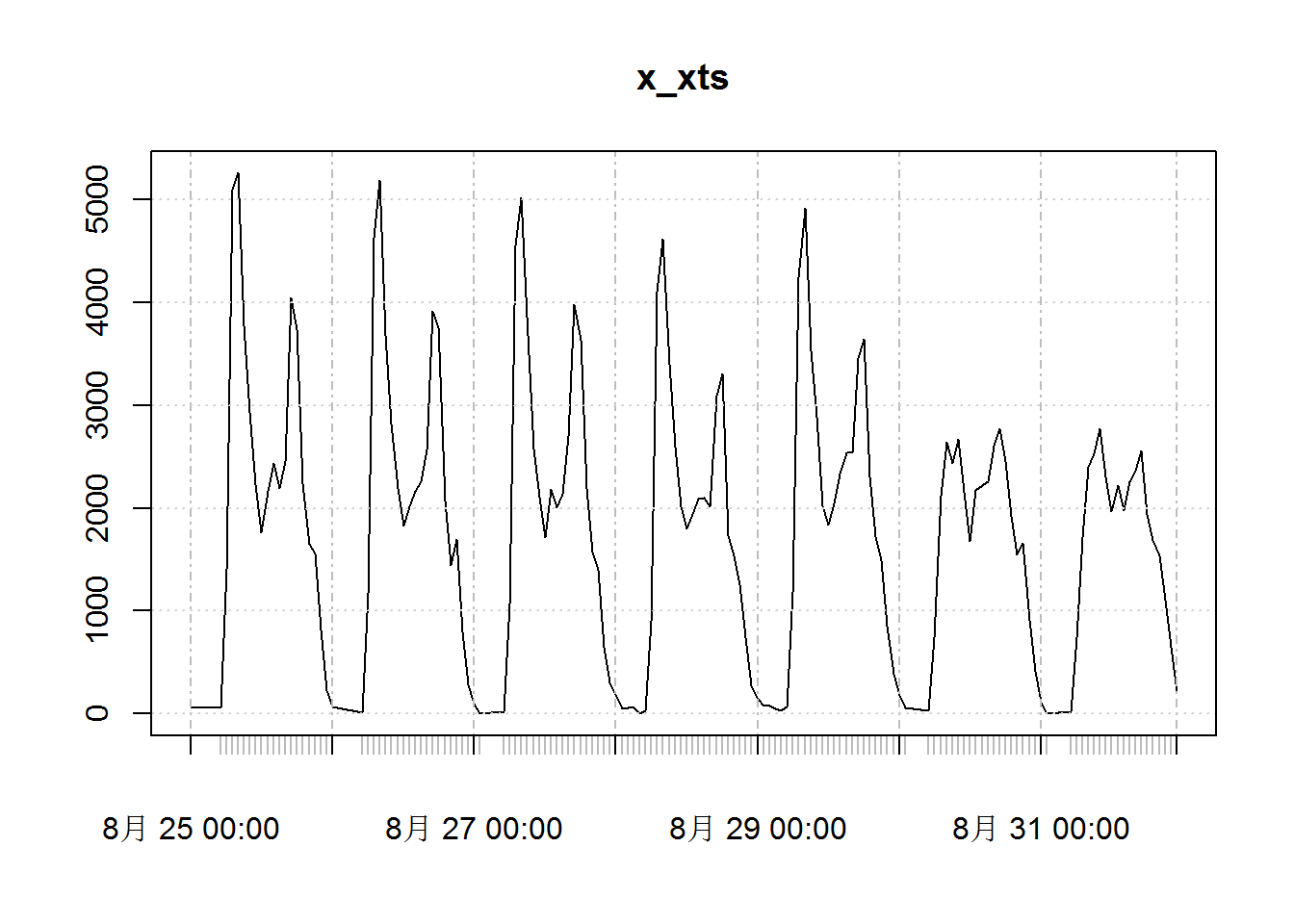xts作为zoo的扩充，提供了一系列针对时间序列相关的函数。

xts is an R package offering a number of functionalities to work on time-indexed data. xts extends zoo, another popular package for time-series analysis.

## 转换成zoo格式

``````x_zoo<-zoo(x,order.by = as.factor(rownames(x)))
plot.zoo(x_zoo)``````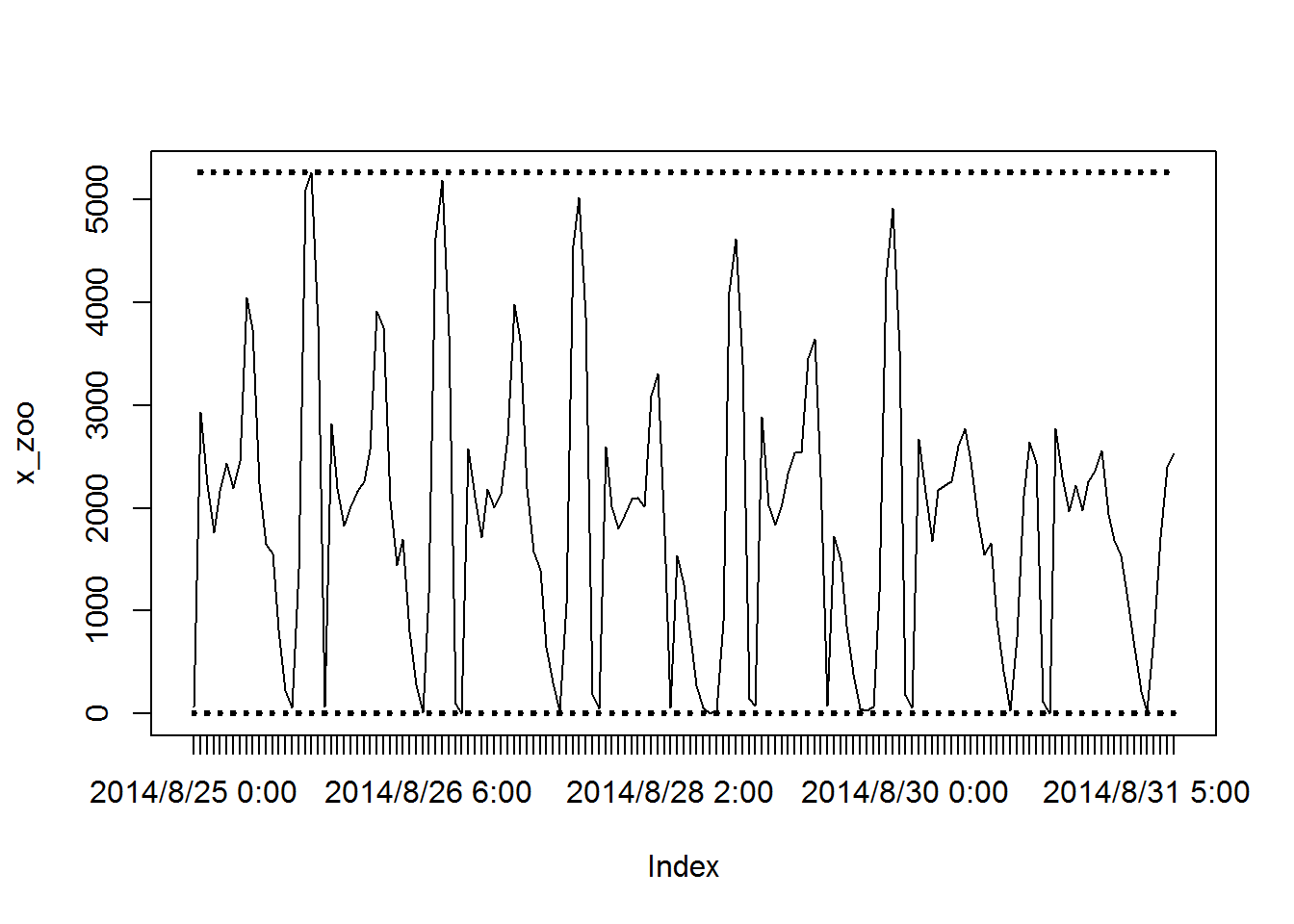## 转换成POSIXct格式

``````x\$time<-strptime(rownames(x), "%Y/%m/%d %H:%M")

## ggplot画图

``ggplot(x,aes(x=time,y=count))+geom_line()``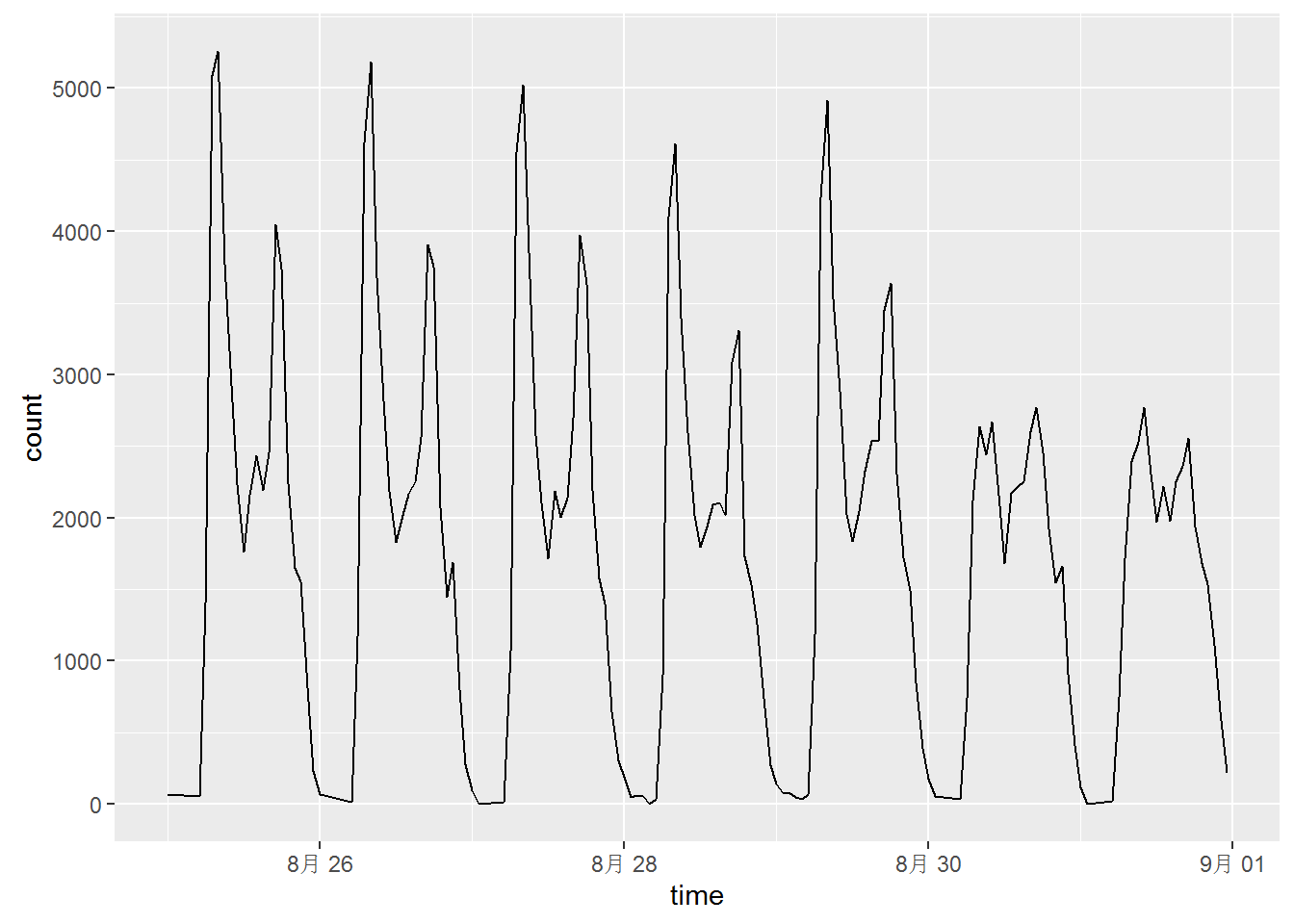### 解决办法一

``````# 参考有关教程得到的方案：

#添加一个新的data.frame
day <- unique(strptime(x\$time,format = "%Y-%m-%d"))
time_df <- data.frame(min=day,
max=day+60*60*24,
fill=as.factor(as.character(day)))

#一定要注意那两个 NULL，少了就会报错。
ggplot(data=x,aes(x=time,y=count))+
geom_rect(aes(NULL,NULL,
xmin=min,
xmax=max,
ymin=min(x\$count),
ymax=max(x\$count),
fill=fill),data=time_df) +
geom_line()``````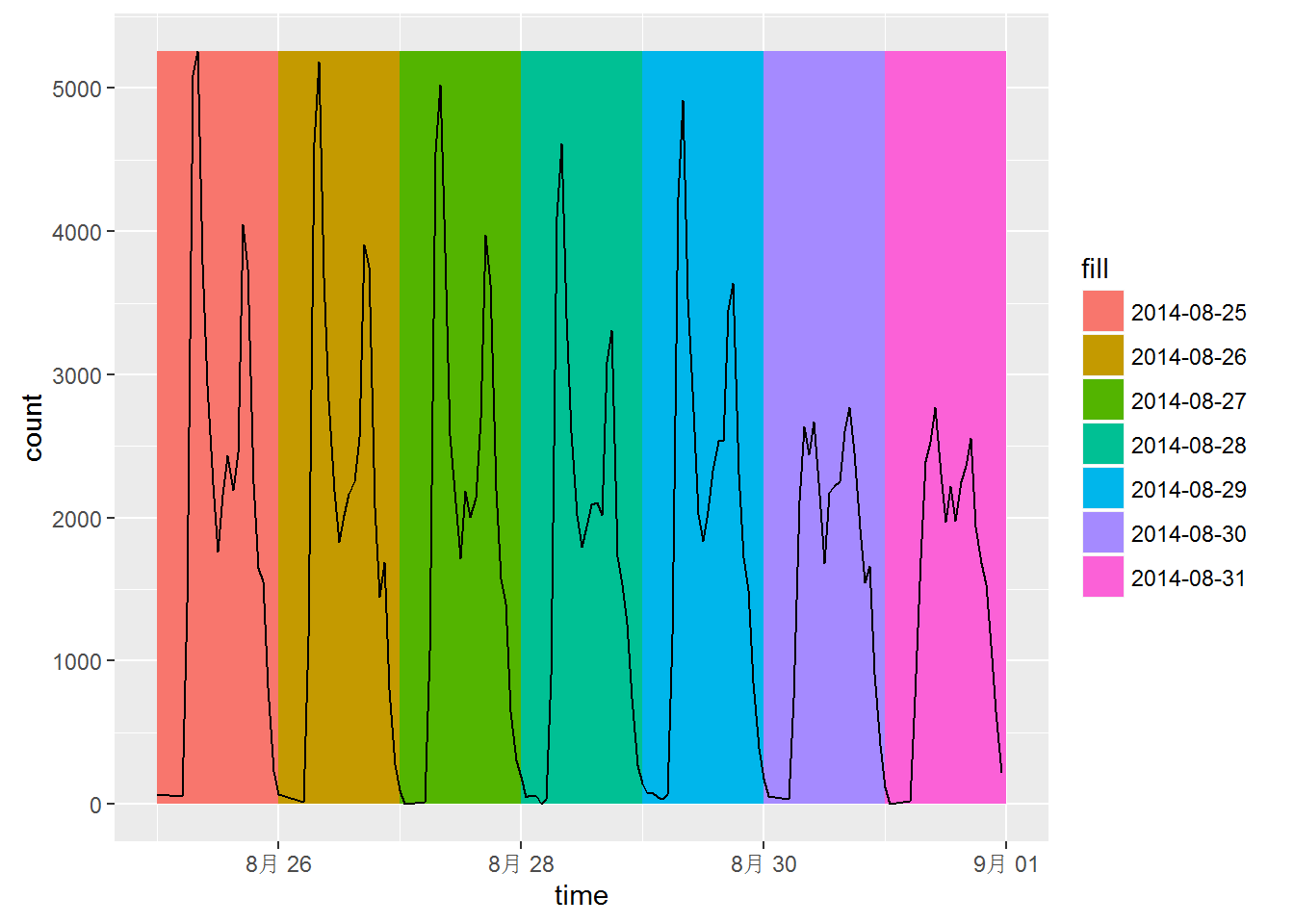``````
#通过上面的例子我相信你已经理解了图层叠加的原理了，
#下面这个例子更直接
ggplot() + geom_rect(data=time_df,
aes(xmin=min,xmax=max,
ymin=min(x\$count),ymax=max(x\$count),
fill=fill),colour="white") +
geom_line(data=x, aes(x=time,y=count))``````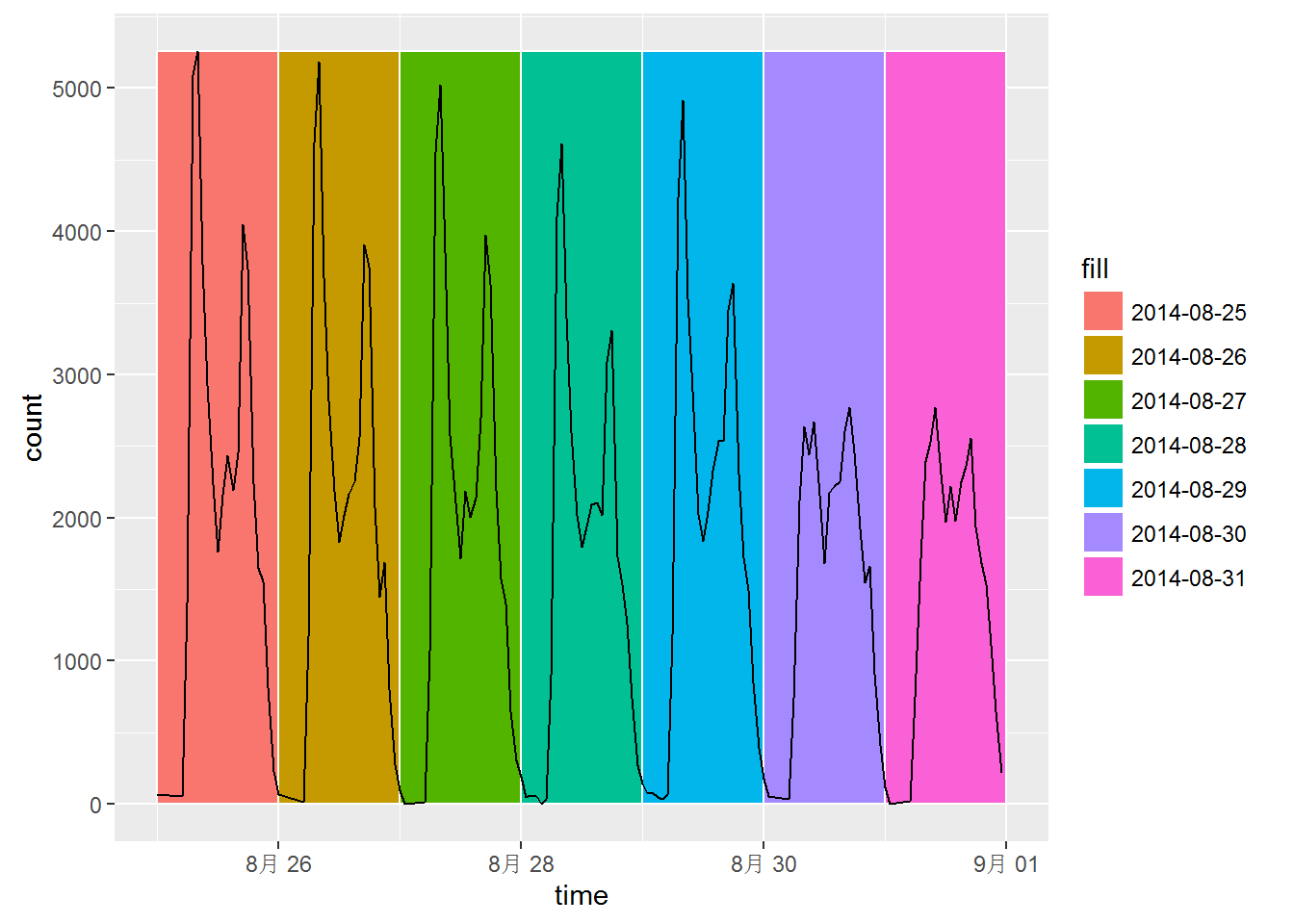### 解决办法二

``````
#对数据整体修改，添加变量
x\$day<-strptime(x\$time,format = "%Y-%m-%d")

#先线再色块， fill 为连续变量,图层叠加原理
ggplot(x,aes(x=time,y=count))+ geom_line()+
geom_rect(aes(xmin=day,
xmax=day + 60*60*24, #一天，单位秒
ymin=min(count),
ymax=max(count),
fill=day),colour="white",alpha=0.1)``````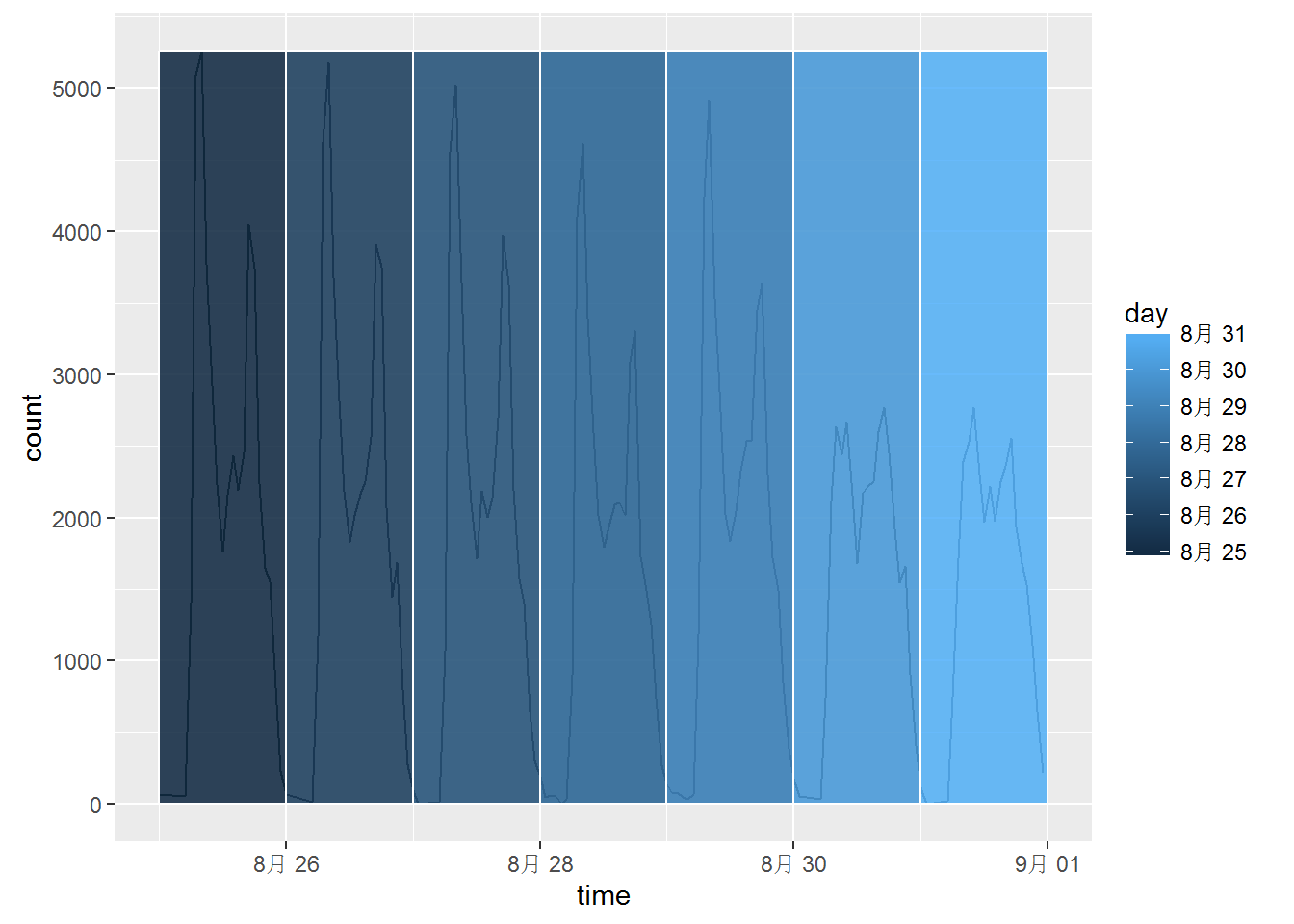``````
#先色块再线 ，fill 为factor
##美化
windowsFonts(myfont=windowsFont("微软雅黑"))

ggplot(x,aes(x=time,y=count)) +
geom_rect(aes(xmin=day,
xmax=day + 60*60*24,
ymin=min(count),
ymax=max(count),
fill=as.factor(as.character(day))),colour="white")+
geom_line() +
labs(title="测试时间格式",fill="日期") +
theme(title=element_text(family = 'myfont',face = "bold"),
text=element_text(family = 'myfont'))``````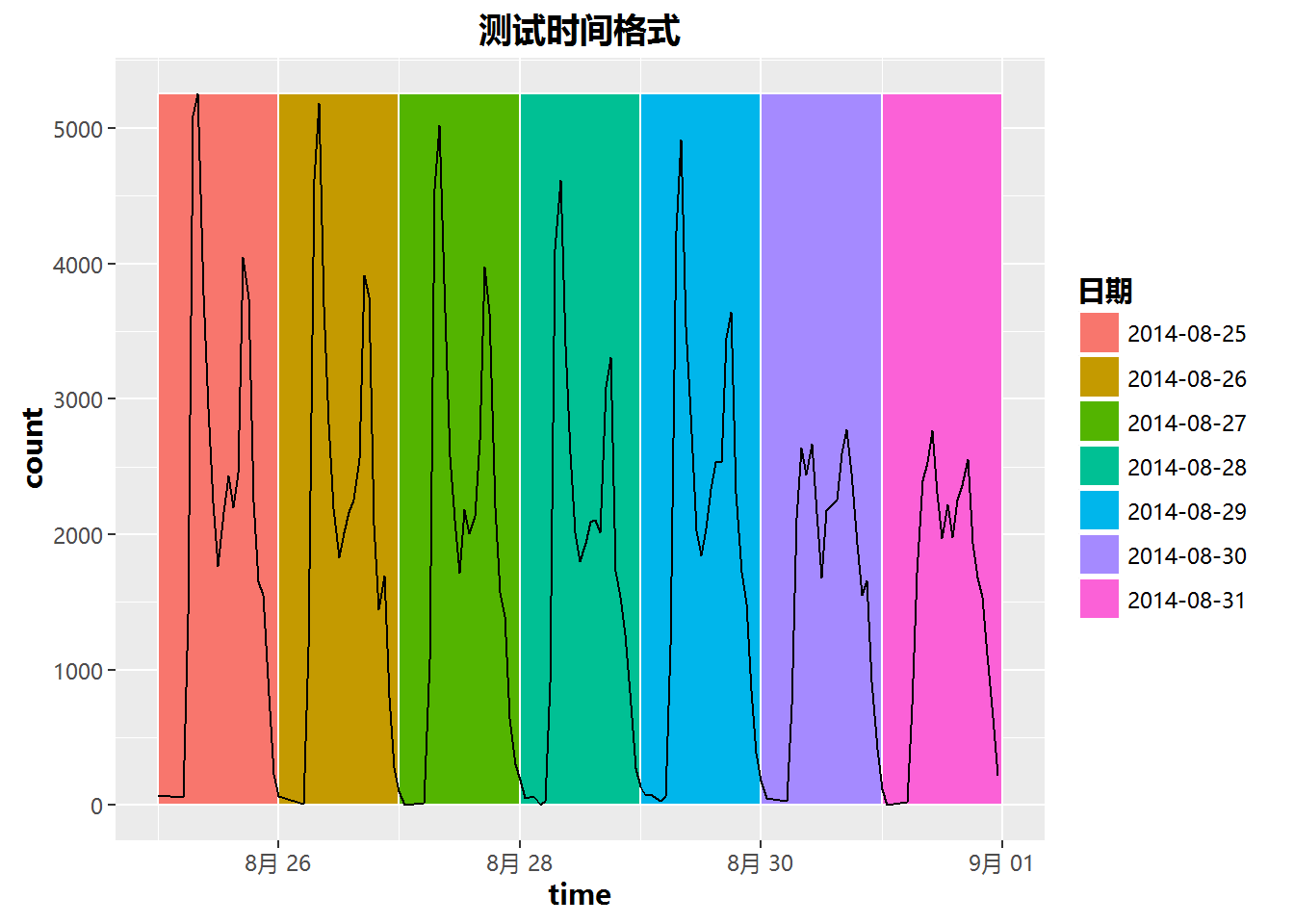## 总结

1. 在用xts或者zoo的时候，碰到了好多坑。下次会专门写一篇处理时间格式的数据
2. 在用ggplot画图的时候，坐标轴的类型一定要是一样的！比如这次横坐标的time是数据类型为`POSIXct`，如果用`as.Date`后的类型的话会报错了
3. 通过这个例子更深入理解了图层语法

## 更新

### 关于调整x轴时间间隔与标签

``````scale_x_datetime(date_breaks = "5 mins",
date_labels = %H:%M",
date_minor_breaks = "1 min")
``````

### 关于zooming

``````# without clipping
coord_cartesian(xlim=c(0,100),ylim=c(10,20))

# with clipping
xlim(0,100) + ylim(10,20)
# or
scale_x_continous(limits=c(0,100) +
scale_y_continous(limits=c(10,20)
``````

Elsa.Liu & Bruce Zhao / -  views
Published under(CC) BY-NC-SA 3.0 CN.

//评论数 //参与数